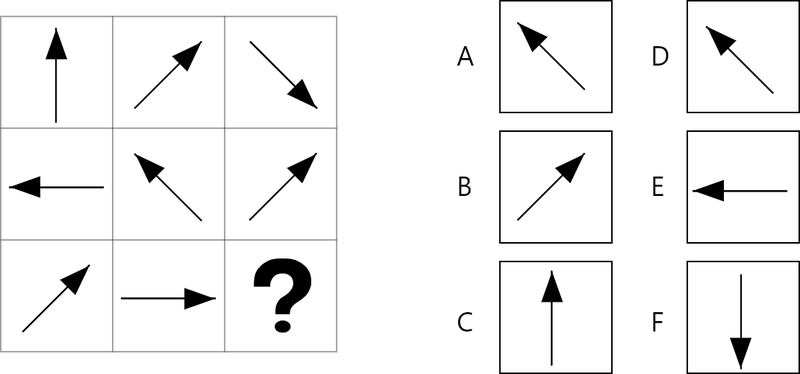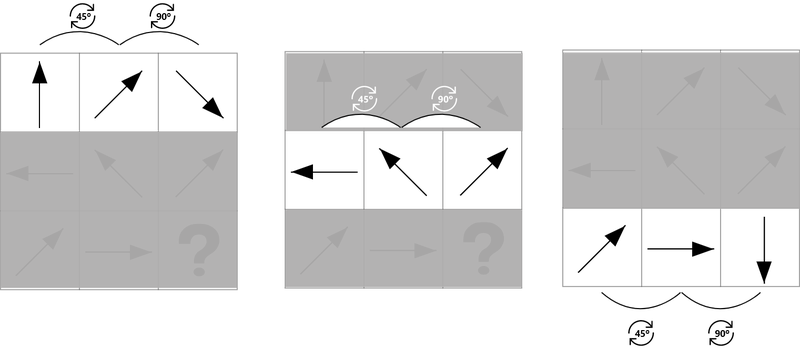# Abstract Matrix Explanation 2 - Change Of Angle Of Figures

#### Matrix explanation - Change of angle of figures

Many of the questions will be (partly) based on the angle (change) of the figures in the boxes. See for example the exercise below:From top to bottom, a clear pattern is not immediately recognizable. From left to right, the arrow first rotates 45 degrees and the next step 90 degrees clockwise. This applies to all rows.The answer is F.

The following transformations are common:

• The same rotation each step, per column or per row
• per row or per column two different rotations (for example, 45 degrees and 90 degrees, as in the example above)
• the rotation increases or decreases per row or column. For example, 45 degrees rotation in the top row, 90 degrees rotation in the middle row and 135 degrees rotation in the last row.
• Each figure points to another figure that moves through the planes.

Tip: Rotations are very common. Look directly at these kind of patterns for each exercise. With some figures such as circles and polygons, a rotation is not (well) visible. You do not have to pay attention to rotation patterns with these figures.